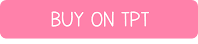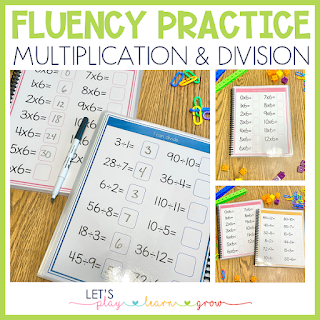# Math Fact Fluency Notebooks

As my kids have gotten older we have found ourselves moving away from our Circle Time activities and more into things we can do at the table together. We still find practicing calendar skills so important so a couple years ago I created our Morning Time Notebooks that we have used and loved during our Morning Time activities. They coordinate so well with our Circle Time Board and can be used alone or together. One of the things I really wanted to incorporate was continued practice with Math facts to help the kids with their math fact fluency. I made an addition and subtraction notebook for Little Dude and a  multiplication and division notebook add-on or Little Miss, both of which can be used separately that we have been using during our Morning Time activities and they have helped my kids tremendously with their math fact fluency.

# Math Fact Fluency Notebooks

Both my kids are very advanced with Math, but one of the things they can use continued practice with is math fact fluency and automaticity.  The more they practice simple math facts, the quicker and easier higher level math is for them. These fluency notebooks are wonderful for morning time, extra math practice, math centers, or quick finisher activities.

## Addition and Subtraction Fluency Notebooks

The addition and subtraction activities can be split into two notebooks or combined into one. Each skill set comes with three different versions of the files. I created them this way so that they could be differentiated for the child and so that you can build fluency with the math facts. The addition and subtraction fluency notebooks includes sums and differences within 20.

### Addition and Subtraction Sets Included:

1. The first set of printables includes all addition/subtraction facts in order. So for instance on the "I can add 1 or subtract 1" page you will see the problems in order: 0+1=, 1+1=, 2+1=, 3+1=, 1-2=, 2-1= etc.
2. The second set of printables includes all addition/subtraction facts out of order. So for instance on the "I can add 2 or subtract 2" page you will see problems like this: 1+2=, 12+2=, 14+2=, 3+2=, 5-2, 7-2=, 12-2=etc.
3. The third set of printables includes mixed addition and subtraction facts combining all number facts together on one page. So for instance on one page you will see: 12+1. 18+1=, 2+10= or 12-2=, 4-1= etc.

## Multiplication and Division Fluency Notebooks

Just like the addition and subtraction activities, the multiplcation and division notebooks can be split into two separate books or combined into one. Each skill set comes with three different versions of the files. The multiplication and division notebook includes facts families through 12.

### Multiplication & Division Sets Included

1. The first set of printables include all multiplication facts or division facts in order. So for instance on the "I can multiply by 1 or I can divide by 1" you will see problems like: 0x1=, 1x1= or 1÷1=, 2÷1= etc.
2. The second set of printables include all multiplication facts or division facts out of order. So for instance on the "I can multiply by 1 or I can divide by 1" page you will see problems like: 5x1=, 3x1=,8x1= or 1÷1=, 12÷1=, etc.
3. The third set of printables include mixed multiplication facts and division facts combining all number facts together on one page. So for instance on one page you will see: 1x1=,2x4=, 12x8 etc.

## Prepping the Notebooks

(These prep resources include Amazon Affiliate links for your convenience, so that you can find and reference the materials easily and quickly. This just means that my blog receives a tiny compensation if you click on these links- at no extra charge to you.)

Each set comes with covers to add to your notebooks. You can have separate addition and subtraction notebooks or combine them into one. The same applies for the multiplication and division notebook. Cover options are available for all 6 choices.

One of my most favorite and most used homeschool prep resources and best investment has been my Proclick Binding machine. I use this to bind everything from printed curriculum to activity books for my kids. The best part is that you can buy the reusable binder spines that open and allow you to swap out what goes in them.

For our Fluency Notebooks, I printed the pages double sided and then laminated them. I used my binding machine to bind them together and then I used the proclick binding spines to bind the books together.

We like to use wet erase markers on our notebooks as it erases easier on the laminating sheets we use, but have also used dry erase markers with success as well (personal preference).

Laminating the pages makes them reusable, but these pages can also be used as printable single use files.

##Bundle and Save

I created everything in my Morning Time series to coordinate together. I don't know about you, but I enjoy when things are cohesive and go together. Both my Morning Time Notebook and Fluency Notebooks follow the same format so they could easily be combined into one notebook or used separately (have have their own cover designs) and they all coordinate with the Circle Time Board.

I bundled all these resources together into two money saving bundles. The first bundle includes just the Morning Time Notebook and Circle Time board and the second bundle is a mega bundle with an even greater discount and it includes the Morning Time Notebook, Circle Time Board, and both Fact Fluency Notebooks.

## Freebie Library

Have you signed up for our Freebie Library yet? Get access to freebies from our free resource library and gain access to sales, discount codes, ideas, and more!!!